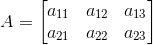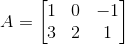Construct a 2 ×3 matrix A = [aj j] whose elements aj j are given by ai j = 2i – j

Asked by Aaryan | 1 year ago |  76

##### Solution :-

Given ai j = 2i – j

Let A = [ai j]2×3

So, the elements in a 2 × 3 matrix are

a11, a12, a13, a21, a22, a23a11 = 2 × 1 – 1 = 2 – 1 = 1

a12 = 2 × 1 – 2 = 2 – 2 = 0

a13 = 2 × 1 – 3 = 2 – 3 = – 1

a21 = 2 × 2 – 1 = 4 – 1 = 3

a22 = 2 × 2 – 2 = 4 – 2 = 2

a23 = 2 × 2 – 3 = 4 – 3 = 1

Substituting these values in matrix A we get,Answered by Sakshi | 1 year ago

### Related Questions

#### Find the values of a, b, c and d from the equations

Find the values of a, b, c and d from the equations:

#### Find x, y, a and b if

Find x, y, a and b if

#### Find x, y, a and b if

Find x, y, a and b if

Construct a 4 × 3 matrix A = [ai j] whose elements ai j are given by $$a_{i j} = \dfrac{(i – j)}{(i + j)}$$